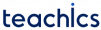# Universal gates (NAND and NOR gate)

The NAND and NOR gates are called universal gates. That is universal gates can be used in combination to perform the AND, OR and inverter operations.

## NAND

The term NAND implies the function of an AND gate with an inverted output. The logic symbol of NAND gate is equivalent to an AND gate followed by an inverter. NAND operation is represented by a bar over the AND expression.

The NAND gate produces a LOW output only when all of its inputs are HIGH. That is for a two-input NAND gate OUTPUT, X is LOW when both inputs A and B are HIGH. X is HIGH if either A or B is LOW, or when both are LOW.

This operation is described in the truth table.

INPUT (A)INPUT (B)OUTPUT (X)
LOWLOWHIGH
LOWHIGHHIGH
HIGHLOWHIGH
HIGHHIGHLOW

### Implementation of Logic Operations Using NAND Gate only

All logic gates can be implemented using NAND gate only.

## NOR

The term NOR implies the function of an OR gate with an inverted output. The logic symbol of NOR gate is equivalent to an OR gate followed by an inverter. NOR operation is represented by a bar over the OR expression.

The NOR gate produces a LOW output when any of its inputs are HIGH. That is for a two-input NAND gate OUTPUT, X is LOW when either input A or B is HIGH or when both A and B is HIGH. X is HIGH only when both A and B is LOW

The operation is summarized in the following truth table.

INPUT (A)INPUT (B)OUTPUT(X)
LOWLOWHIGH
LOWHIGHLOW
HIGHLOWLOW
HIGHHIGHLOW

### Implementation of Logic Operations Using NOR Gate only

Since NOR gate is a universal gate, its combination can be used to implement any other logic operation.

#### NAND using NOR gate only